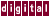# A.2 Varieties of Data Dependence

The following describes three kinds of data dependence. The notation `S1, S2,` and `SN` is used to denote statement 1, statement 2, and statement N, respectively. In each example, `S2` is dependent on `S1` , due to variable `x` .

1. Data dependence from an assignment to a use of a variable is called flow dependence or true dependence.
```(S1): x = 3;
(S2): y = x;
```

2. Data dependence from use of a variable to a later reassignment of that variable is called antidependence.
```(S1): y = x;
(S2): x = 3;
```

3. Data dependence from an assignment of a variable to a later reassignment of that variable is called output dependence.
```(S1): x = 3;
:
(SN): x = 4;
```

Previous Page Next Page Contents Index
Command-Line Qualifiers